# HBSE 9th Class Science Important Questions Chapter 10 Gravitation

Haryana State Board HBSE 9th Class Science Important Questions Chapter 10 Gravitation Important Questions and Answers.

## Haryana Board 9th Class Science Important Questions Chapter 10 Gravitation

Question 1.
What is meant by gravitation ?
Everybody on earth attracts each other. Such type force of attraction between bodies is called gravitation.

Question 2.
Who discovered the gravitational force firstly ?
Sir Isaac Newton.

Question 3.
In which direction the gravitational force between two objects works ?
The gravitational force between two objects works in the direction of line joining the centre of their masses. .

Question 4.
Which force is responsible for the existence of our solar system ?
Gravitational force is responsible for our solar system.

Question 5.
What ‘G’ indicates ?
‘G’ indicates gravitational constant.

Question 6.
What is the value of ‘G’ ? ’
VMtte of‘G’ is 6.673 x 1011 Nm2/kg2

Question 7.
How much is the mass of earth ?
Mass of earth (Me) = 6 x 1024 kg (approx.).

Question 8.
How much is the radius of earth ?
Radius of earth (Re) = 6.4 x 1o6m.Question 9.
At which part of earth, the value of g is maximum and minimum?
The value of g is maximum on poles of earth and minimum on the equator. ‘

Question 10.
Write the formula for the force of attraction between two objects of masses m1 and m2 respectively kept at a distance of R.
F = $$\frac{\mathrm{Gm}_1 \mathrm{~m}_2}{\mathrm{R}^2}$$

Question 11.
What is acceleration due to gravity (g) ?
The acceleration by which earth attracts other object towards its centre is called acceleration due to gravity.

Question 12.
Does the acceleration of an object falling towards earth depend upon its mass ?
No, the acceleration of an object falling towards earth does not depend upon its mass.

Question 13.
What will be the force of attraction between two objects if the distance between them becomes twice ?
Force of attraction will become one-fourth.

Question 14.
What will be the weight of an object on moon in comparison to earth ?
$$\frac {1}{6}$$ times.

Question 15.
What is centripetal acceleration ?
When an object moves on a circular track’ ah acceleration is produced due to change in velocity, which is called centripetal acceleration.

Question 16.
What is centripetal force ?
The force which produces centripetal acceleration is called centripetal force. It is always directed towards centre. That is, F = $$\frac{\mathrm{mv}^2}{\mathrm{r}}$$

Question 17.
What ‘g’ indicates ?
‘g’ indicates acceleration due to grayity.

Question 18.
What is the value of ‘g’ near the syrfepe of earth ?
The value of ‘g’ is 9.8 m/s2.

Question 19.
On which place, the value of ‘g’ is zero ?
The value of‘g’is zero at the centre of earth.

Question 20.
Write the formula for the relation between ‘g’ and ‘G’
g = $$\frac{\mathrm{G} \times \mathrm{M}_{\mathrm{e}}}{\mathrm{R}^2}$$Question 21.
What are the factors on which the gravitational force depends ?
(i) On the mass of objects.
(ii) On the distance between objects.

Question 22.
What is the distance of moon from earth ?
60 times of the radius of earth – 384000 km.

Question 23.
If an object is dropped from height A with zero velocity, then how much distance it will cover in one second ?
Distance covered, h = $$\frac {1}{2}$$ gt2

Question 24.
Whose value, out of ‘g’ and ‘G’ is constant and whose not ?
The value of ‘G’ is constant at every place on earth, but value of ‘g’ is more on poles and less on equators.

Question 25.
How much time does the earth take to move around the sun ?
The earth takes 365 $$\frac {1}{4}$$ days to move around the sun.

Question 26.
How much time does the moon take to move around the earth ?
The moon takes 27 days 8 hours to move around the earth.Question 27.
What is meant by mass ? Write its unit.
Mass is the quantity of matter contained in it. Its unit is kilogram.

Question 28.
What is meant by weight ? What is its measurement unit ?
The force by which earth attracts an object towards itself is called the weight. Its measurement unit is Newton.

Question 29.
What is meant by weight lessness ?
The state of body when gravity of planet (earth) stops acting, the person in spaceship is in the state of weightlessness.

Question 30.
What is meant by centre of mass ?
The centre of mass is that point at which whole mass of object is directed.

Question 31.
What is meant by centre of gravity ?
The centre of gravity is that point of the object at which the whole force of gravity works.

Question 32.
Where is the centre of gravity of objects having regular shape ?
The centre of gravity of die objects having regular shape and equal density lies on geometrical centre.

Question 33.
What will happen if the mass and acceleration of two objects become equal ?
If the mass and acceleration of two objects become equal, then they will appear to move towards ‘ each other.Question 34.
What is meant by the force of gravity ?
The force between earth and an object is called the force of gravity.

Question 35.
Which balance is used to measure weight ?
Spring balance is used to measure weight.

Question 36.
Which balance is used to measure mass ?
Physical balance is used to measure mass.

Question 37.
Why is the weight of the object less on the moon in comparison to the earth ?
Because the acceleration due to gravity on the ftibon is less than that on the earth.

Question 38.
Two stones of masses 25 kg and 2 kg are dropped at the same time from the top of a building. Which stone will reach first on earth ?
The acceleration due to gravity does not depend upon the mass of the object. So both stones will reach on the earth in same time.

Question 39.
There is gravitational force between two objects kept on table. Why they don’t move towards each-other even in the presence of this force ?
The gravitational force between two objects is very weak so that they do not move.

Question 40.
The earth attracts an iron pin downward with gravitational force, but the magnet attracts the iron pin upwards against the direction of attraction of earth, why ?
Because attractive force applied by magnet on iron pin is more than the gravitational force on pin.

Question 41.
Where the gravitational force will be more-between two objects on earth or between object and earth ?
The gravitational force between object and earth is more.Question 42.
Where is the weight of the object zero ?
The weight of the object is zero at the centre of earth.

Question 43.
What is the relation among u, v, g and t ?
v = u + gt

Question 44.
What is the sign of ‘g’ according to Cartesian Coordinate system ?
‘g’ is always taken as negative.

Question 45.
What is meant by projectile range ?
The maximum horizontal distance covered by projectile is called projectile range.

Question 46.
What is the angle between velocity and acceleration at the highest point of projectile ?
Right angle (90°).

Question 47.
How far is the sun from the earth ?
1.5 x 1011 metres.

Question 48.
What is meant by thrust ?
The force acting perpendicular to the surface of object is called thrust.

Question 49.
Give an example of thrust in daily life.
When we press a pin on drawing board, the force applied by our thumb acts in perpendicular direction on board. Thus, it is thrust.

Question 50.
What is pressure ? What is its SI unit ?
The thrust per unit area is called pressure. That is pressure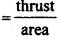Its SI unit is Pascal (Pa).

Question 51.
What is the SI unit of thrust ? Africa
Newton (N).

Question 52.
In regard of which scientist, the SI unit of pressure is Pascal (Pa) ?
In honour of scientist Blaise Pascal.

Question 53.
What is the bigger unit of pressure ?
Kilopascal (k Pa).Question 54.
Why cutting, tearing and grooving apparatus are always sharp ?
The cutting, tearing and grooving apparatus are sharp so that thrust affects minimum area to increase pressure and the object can be cut, tear or groove easily.

Question 55.
When is the pressure of man more -sitting on ground or standing at one place and why ?
The pressure of man is more when standing at one place because the area in standing position is less than that in sitting position at one place.

Question 56.
What is the effect of depth on liquid pressure ?
The liquid pressure increases with depth.,

Question 57.
Why do the egg floats in salty water ?
The density of water increases by adding salt in it, due to that buoyant force of water increases and the egg floats.

Question 58.
In which stage, the object floats ?
If the weight of the body is equal to the weight of fluid displaced, then the object floats on the surface of fluid.

Question 59.
Write the name of two devices that work on Archimedes’ principle.
(i) Ship and
(ii) submarine.

Question 60.
Which device is used to measure the purity of the milk and what is its principle ?
The lactometer is used to measure the purity of the milk which works on Archimedes’ principle.

Question 61.
Write the name and principle of that device which is used to measure the density of liquids.
Hydrometer, which works on Archimedes’ principle.

Question 62.
What is the mass of unit volume called ?
Density.

Question 63.
What is the SI unit of density ?
Kilogram per metre cube (kg m-3).Question 1.
If a piece of stone is allowed to fall freely from a certain height, then what will be the changes in velocity ?
The following are the changes :
(1) The initial velocity of stone is zero and it increases at coming down.
(2) The stone nearest to earth has maximum velocity.
(3) The velocity of stone becomes zero after striking the ground. –

Question 2.
What is gravitation ? In which direction it works ?
Everybody of universe attracts other body towards itself. This attraction between two bodies is gravitation. This attraction between two bodies works along the line joining the centres.

Question 3.
Prove that acceleration produced in the object dropped towards the earth does not depends upon its mass.
If an object of mass, m is dropped from the centre of earth at a distance of R, then the force exerted by the earth on object,
F = $$\frac{\mathrm{GM}_{\mathrm{e}} \mathrm{m}}{\mathrm{R}^2}$$
Where, Me = mass of earth, G = gravitation constant
∴ Acceleration produced in the object = $$\frac {F}{m}$$ (F = ma; a = $$\frac {F}{m}$$)
= $$\frac{\frac{\mathrm{GM}_{\mathrm{e}} \mathrm{m}}{\mathrm{R}^2}}{\mathrm{~m}}$$ = $$\frac{\mathrm{GM}_{\mathrm{e}} \mathrm{m}}{\mathrm{R}^2} \times \frac{1}{\mathrm{~m}}=\frac{\mathrm{GM}_{\mathrm{e}}}{\mathrm{R}^2}$$
∴ The acceleration produced in an object does not depend upon the mass of dropped object.

Question 4.
What is Newton’s third law of motion ? Is this law applicable on gravitational force ? Explain.
According to Newton’s third law, “To every action, there is equal and opposite reaction.” For example, we act on ball when we throw it on wall and ball comes back by the reaction of wall. Thus, an object A exerts a force on object B, then object B is also exerts equal and opposite force on A. This fact is also true for gravitational force. When a stone falls on earth, it happens due to the force of gravity of the earth on stone. In fact, the piece of stone, attracts the earth towards itself with equal force of gravity. But the mass of stone is less than that of the earth so the force exerted by the stone on the earth is less. Therefore the stone seems to attracted towards earth.Question 5.
Discuss the motion of the object thrown upward.
When a body is thrown upward, the earth attracts that body continuously towards itself. Therefore, the gravitational force works in opposite direction of motion and the body moves slowly. The speed reduces at a rate of 9.8 ms-2 in every second. At last when the body reaches at highest point, the speed becomes zero. The body again starts falling towards earth with an acceleration of 9.8 ms-2.

Question 6.
Find the unit of ‘G’
‘G’ is the ratio of force (F) and $$\frac{M_1 M_2}{R^2}$$ Therefore, its unit depends upon the units offorce, mass and distance. If the mass is taken in kilogram, distance in metre and time in second, then the uqit offorce is newton (N).
Unit of G = $$\frac{M_1 M_2}{R^2}$$ = Nm2/kg2

Question 7.
Why is G called universal constant ?
We know that F = G $$\frac{m_1 m_2}{R^2}$$
‘G’ is constant among diem. The value of ‘G’ does not depend upon the Value of mj, m2 or R. Its value does not depend upon that how, when and where the force is measured. The value of‘G’ remains consant for any two bodies in universe. The ratio of F and $$\frac{m_1 m_2}{R^2}$$ is equal to G which is equal for any two bodies. That is why, G is called universal constant.Question 8.
What is the difference between ‘g’ and ‘G’ ?
The difference between ‘g’ and ‘G’ is as follows:

 g: G: 1. It indicates acceleration due to gravity. 1. It indicates gravitational constant. 2. Its value vary from place to place. 2. Its value is same everywhere. Therefore, it is called universal constant. 3. Its value on earth is 9.8 ms-2. 3. Its value is 6.673 x 10-11 Nm2 kg-2. 4. It is always negative. 4. It is always positive.

Question 9.
Prove that the bodies of different masses when dropped together from the same height, strikes in same time (assume, air resistance is negligible).
S0lution:
Suppose two objects of different masses were dropped together from same height h,
In this state s = h, u = 0
According to formula s = ut + $$\frac {1}{2}$$ gt2
h = 0 x t + $$\frac {1}{2}$$ gt2
Or h = $$\frac {1}{2}$$ gt2
Or 2h = gt2
Or gt2 = 2h
t2 = $$\frac {2h}{g}$$
Or t = $$\sqrt{\frac{2 h}{g}}$$
It is clear from the equation that the time taken by objects does not depend upon masses. Thus, both objects fall in same time on earth.Question 10.
What is meant by vertical direction of earth?
If we suppose the earth as a ball of uniform density, then its centre of mass will be at its centre. Thus, the force of attraction of the earth on a body works in the direction of its (earth) centre. This is the direction which we call vertical direction.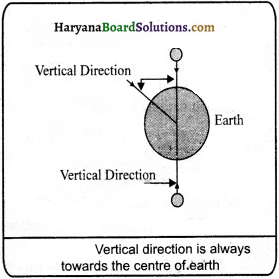Question 11.
Which are equations related to the freely falling bodies?
Solution:
(i) v = u + gt
(ii) v2 – u2 = 2gs
(iii) s = ut + $$\frac {1}{2}$$ gt2
Here,
u = initial velocity
y = final velocity
g = acceleration due to gravity
s = distance or height
t = time

Question 12.
What is meant by weight ? Write the formula for weight.
The force of attraction applied by earth on an object is called its weight. if earth produces an acceleration ‘g’ on an object of mass, m then, the force of artraction applied by earth on object, F = mg
But, the force of attraction applied by the earth on object (F) = Weight of object (W) = W = mgQuestion 13.
Find a formula to compare the weight of a body on earth and on any other heavenly body.
Solution:
Suppose, mass of the object = m
Mass of the earth = Me
The attractive force applied by earth on object, Fe = $$\frac{\mathrm{GM}_{\mathrm{e}} \mathrm{m}}{\mathrm{R}_{\mathrm{e}}{ }^2}$$ ………..(i)
Suppose, mass of hçavenly body = Mm
Radius of heavenly body = Rm
The force of attraction applied by other heavenly body on object
Fm = $$\mathrm{G} \frac{\mathrm{M}_{\mathrm{m}} \times \mathrm{m}}{\mathrm{R}_{\mathrm{m}}^2}$$ …………..(ii)

Dividing eq (ii) by eq (1)
$$\frac{\mathrm{F}_{\mathrm{m}}}{\mathrm{F}_{\mathrm{e}}}=\frac{\mathrm{M}_{\mathrm{m}}}{\mathrm{M}_{\mathrm{e}}} \times\left(\frac{\mathrm{R}_{\mathrm{e}}}{\mathrm{R}_{\mathrm{m}}}\right)^2$$

Question 14.
Find the relation between acceleration due to gravity (g) and universal gravitational constant (G).
Suppose an object of mass m is kept at a distance of R from the centre of the earth. If M is the mass of the earth, then the force applied by earth on that object:
F = $$\mathrm{G} \frac{\mathrm{Mm}}{\mathrm{R}^2}$$ ………..(i)
The force by which the earth attracts the object towards its centre, is cal led weight. It is also denoted by’ F’.
F = mass (m) x acceleration due to gravity (g)
or F = mg ……….(ii)
From equations (i) and (ii) mg = $$\mathrm{G} \frac{\mathrm{Mm}}{\mathrm{R}^2}$$
Or g = $$\frac{\mathrm{GM}}{\mathrm{R}^2}$$
This is the required relation. The value of’g’ can be found with the help of this.

Question 15.
What is mass ? What is its measurement unit ? WrIte the law to measure mass.
Mass: The quantity of matter contained in an object is called its mass. It is a scalar and constant quantity.
The unit of mass is kilogram. It is found by physical balance.

Law to measure mass:
Suppose we have two objects of masses m1 and m2, kept at the same distance from the centre of the earth, then the force of attraction between the earth and m1:
F1 = R2
Here, Me = mass of earth and R = distance of mass m1 from the centre of the earth.
Like this, the force of attraction between the earth and m2
F1 = $$\frac{\mathrm{GM}_{\mathrm{e}} \mathrm{m}_1}{\mathrm{R}^2}$$
Here, Me = mass of earth and R = distance of mass m1 from the centre of the earth.
Like this, the force of attraction between the earth and m2
F2 = $$\frac{\mathrm{GM}_{\mathrm{e}} \mathrm{m}_2}{\mathrm{R}^2}$$
1f both the forces are same, then F1 = F2
$$\frac{\mathrm{GM}_{\mathrm{e}} \mathrm{m}_1}{\mathrm{R}^2}=\frac{\mathrm{GM}_{\mathrm{e}} \mathrm{m}_2}{\mathrm{R}^2}$$
∴ m1 = m1
Thus, if two forces are equal then the masses of two objects will be equal and parts of physical balance will be equal.Question 16.
Define projectile and path of projection and give some examples of projection motion.
Projectile:
A body thrown in horizontal direction is called projectile.

Path of projection:
The curved path travelled by projectile is called path of projection.

The examples of projection motion are following:
(1) The path of a body dropped from aeroplane, i.e., a ball falling from a building of 80m high is shown in figure.
(2) The path of a bullet fired from gun.
(3) The path of sphere thrown by player.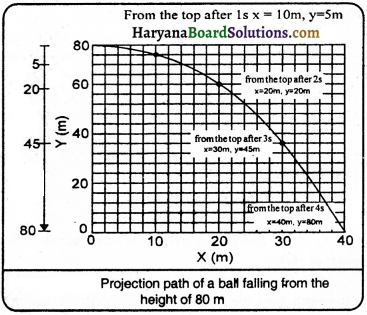Question 17.
Why do astronauts feel weightlessness in spaceship?
When the astronauts sitting in spaceship move around the earth, the gravitational force between the earth and spaceship is balanced by the force used to move around the earth, due to that the astronauts feel weightlessness. The value of acceleration due to gravity becomes zero in spaceship.

Question 18.
Generally, if the cotton ball and stone of same size are allow ed to fall from a certain height, then which one will strike the surface of earth first? What Galello discussed about this fact?
Generally, the piece of stone reaches first on the surface of earth in comparison to cotton. But first of all Galelio told that the air resistance becomes zero, cotton ball and piece of stone will reach in same time on the surface of earth with equal acceleration. According to Galelio. “the objects the acceleration of falling on the acceleration of the earth does not depend upon their masses.”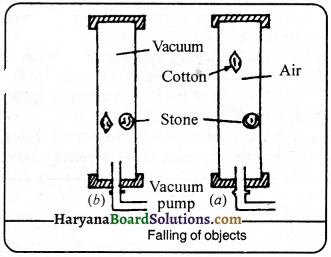Question 19.
How does acceleration due to gravity change at any place (i) due to height, (ii) due to the shape of the earth?
In the following way the acceleration due to gravity changes at any place:
(i) Due to height: The value of ‘g’ is less on the mountains situated at some height on earth surface, whereas it ¡s more on plain surface.
∴ $$\frac{W_h}{W}=\frac{g_h}{g}=\frac{R^2}{(R+h)^2}$$
Like this, the value of ‘g’ goes on decreasing by increasing height.

(ii) Due to the shape of earth: The earth is not completely round like a ball, but it is convexed at the equator line and concaved at the polar line. There is a difference of 22 km between the radius of the equator and polar line of the earth. That’s why
the value of’g’ is more on poles and less on equators.
∴ g = $$\frac{\mathrm{GM}}{\mathrm{R}^2}$$ (Where, G and M are constant.)
∴ g ∝ $$\frac{1}{R^2}$$Question 20.
“The moon falls towards the earth.” What does it mean ? Why does it not fall on the surface of the earth?
The moon falls towards the earth means that the earth attracts the moon towards itself due to its gravitational force. But, moon moves around the earth in a circular path and does not fall, because the velocity of the object moving on a circular path changes at every point. The change in velocity or acceleration is due to the change in the direction of motion of the object. The centripetal force which produces this acceleration maintains the body to move in circle, which is always directed towards the centre.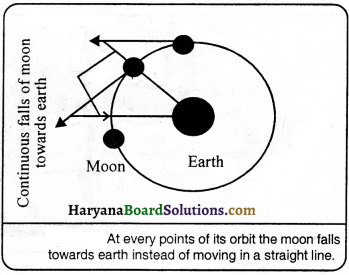Question 21.
The earth attracts the apple, does the apple also attract the earth ? If yes, then why the earth does not move towards the apple?
Definitely the apple attracts the earth towards itself, but the mass ofapple is negligible in comparison to earth so the earth does not move towards apple.

Question 22.
What are Kepler’s three laws of motion?
Kepler’s is three laws of motion are following:
(i) The orbit of a planet is an ellipse with the sun at one of the foci. As the position of sun is shown by in the figure.
(2) The line joining the planet and the sun sweep equal area in equal intervals of time. Thus, if the time of travel from A to B is same as that from C to D, then the areas OAB and OCD are equal.
(3) The cube of the mean distance of a planet from the sun is proportional to the square of its orbital period, T, or constant. It is important to know that Kepler could not give a theory to explain the motion ofplanets. It was Newton who showed that the cause of the planetory motion is the gravitational force that the sun exerts on them.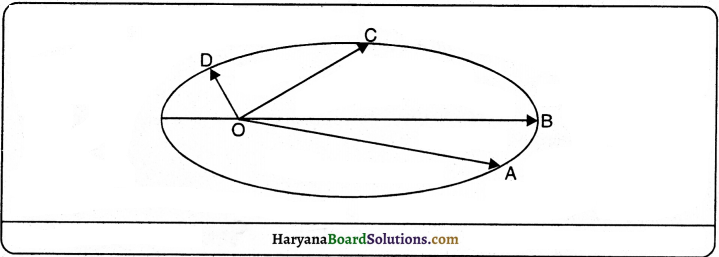Question 23.
Why are the tyres of buses or trucks wider than that of cars?
The thurst on buses or trucks is more than that on cars. If their tyres are made less wider than the tyres of cars, the pressure on them will increase and they will sink ¡n earth. Therefore, the tyres of buses or trucks are made wider, so that the area will increase and pressure on earth decrease.Question 24.
The camel can walk in desert easily, but ¡t is difficult for horse to walk, why?
The area of feet of camel is more than that of horse. So the affected area is more and pressure decreases. The camel can walk easily in desert due to less pressure. But the area of feet of horse is less, and the pressure increases. Due to large pressure, it is difficult to walk by horse in desert.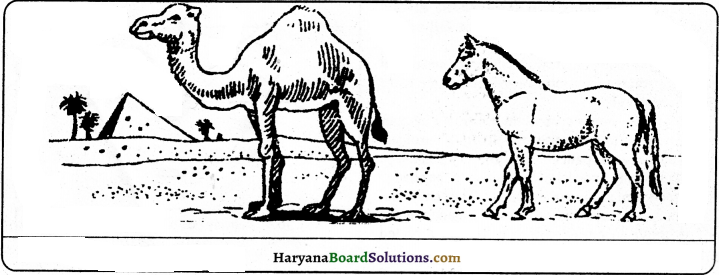Question 25.
What is buoyant force? [low is it measured?
When a body is immersed in a fluid, an upward force works on the body which is called buoyant force. Measurement of Buoyant Force: Take a metal’s sphere. Hang it on spring balance and note its weight. Let its weight is W1 gm. Now put some water in a beaker and take the sphere in the beaker slowly. You will see that the weight of sphere seems decreasing when put in water slowly, which is indicated by spring balance. Note the indicator of spring balance when the solid sphere immersed completely in water.
Let it is W2 gm.
The difference between two weights will be the buoyant force of water.
Buoyant force = weight of sphere in air – weight of sphere in water.
= (W1 – W2)gm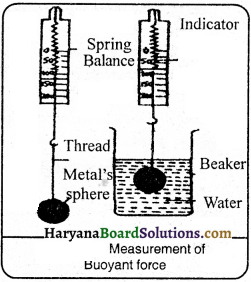Question 26.
What is the reason that, an iron needle sinks in water, but a ship made up of iron floats in water?
The iron needle sinks in water, but a ship made of iron floats in water because weight of water displaced by needle is less than the weight of needle. Due to heavy weight, the needle sinks in water. But the weight of water displaced by ship is more than the weight of ship and it floats in water.

Question 27.
Why is it easy to swim in sea water than river?
It is easy to swim in sea water than river because the density of sea water is more than the density of river water. Thus, the sea water due to being heavy, applies larger buoyant force on the body of person, duc to this reason it is easy to swim in sea water.

Question 28.
What is density ? What is its SI unit ? What is its importance?
The mass per unit volume of a body is called density. Its SI unit is kilogram per metre cube. The density of the substance always remain same in certain circumstances. The density of gold ¡s 19300 kg m-3 and the density of water is 1000 kg m-3.
Importance: The density of the sample of any substance helps in the measurement of its purity.

Question 29.
What is relative density ? What is the condition of sink or float in water on the basis of it?
The ratio of density of substance and density of water is called relative density of the body. Thus density of substance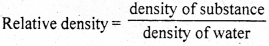∴Relative density is a ratio, so, it has no unit.
The relative density ofgold is 19.3. If the relative density of any solid or liquid is more than I, it will sink in water, if it is less than 1, it will float in water.Question 30.
Why the ice floats on water?
The ice floats on water because the density of ice is less than the density of water. when a piece of the ice is put in the water, then its weight is less than the weight of water displaced by it.

Question 31.
Define Archimedes’ principle and explain it. What arc uses of this principle? Or Define Archimedes’ Principle. Write Iwo applications ofArchimedes’ Principle.
Statement of Archimedes’ principle: When a body is immersed fully or partially ¡n a fluid, it expirences an upward force that is equal to the weight of the fluid displaced by it.

Explanation:
When a solid is immersed in a fluid, the fluid pushes it upwards by its buoyant force, and its wekht decreases, because buoyant force is equal to the weight of water displaced by the solid. So the decrease in weight of solid when immersed ¡n fluid is equal to the weight of the water displaced.

Uses of Archimedes’ principie in daily life:
(I) It is used in designing of ships and submarines.
(2) The lactometer, which is used to determine the purity of milk, is based on this principle. .
(3) The hydrometer which is used for determining density of liquid, is also based on this principle.

Question 32.
What happens to the gravitational force between two objects If:
(i) the distance between the objects ¡s one-third (1/3)?
(ii) the masses of both the objects are halfed (1/2)?
(i) As we know that gravitational force between two objects is inversely proportional the square of the distance between them. So, if the distance between them is then the gravitational force between them will be 9 times.
(ii) As the gravitational force between two objects is proportional to the product of masses of two objects. So, if the masses of two objects are halfed (1/2) then the gravitational force between them will be one tourth (1/4).

Numerical Questions

Question 1.
A bullet is fired with a velocity of 25 ms1 in horizontal direction. How much distant it will fall in first two seconds?
Solution:
Vertical distance is independent to horizontal velocity.
∴ Initial velocity (u) = 0
Acceleration due to gravity (g) = 9.8 ms-2
Time (t) = 2s
Distance (s) = ?
We know that
s = ut + $$\frac {1}{2}$$ gt2
= 0 x 2 +$$\frac {1}{2}$$ x 9.8 x (2)2
= 19.6 m

Question 2.
Find the weight of an object of mass 5 kg.
Solution:
Mass of the object (m1) = 35 kg
Acceleration due to gravity (g) = 9.8 ms-2
Weight of the object (W) = ?
We know that, W = m x g = 5 x 9.8 = 49 NQuestion 3.
If the mass of you and your friend is 35 kg, and the distance between you and your friend is 1 m, then what will be the force of attraction between two?
Solution:
Here,
Mass(m1) = 35kg
Ma.ss(m2) = 35kg
Distance(r) = 1 m
Gravitational constant (G) = 6.67 x 10-11 Nm2 kg-2
Force of attraction (F) = ?
We know that, Force of attraction, (F) = $$\frac{\mathrm{Gm}_1 \mathrm{~m}_2}{\mathrm{r}^2}$$
= $$\frac{6.67 \times 10^{-11} \times 35 \times 35}{(1)^2}$$
= 8.17 x 10-8 N Ans

Question 4.
FInd the weight of an object of mass 10 kg on the surface of that planet whose mass is two times and radius Is three times that of earth.
Solution:
For planet, M = 2Me and R = 3 Re
∴ g1 = $$\frac{\mathrm{G}\left(2 \mathrm{M}_{\mathrm{e}}\right)}{\left(3 \mathrm{R}_{\mathrm{e}}\right)^2}=\frac{2 \mathrm{GM}_{\mathrm{e}}}{9 \mathrm{R}_{\mathrm{e}}^2}$$
= $$\frac {2}{9}$$ g
= $$\frac {2}{9}$$ x 9.8 = $$\frac {19.6}{9}$$m-2 [∵ g = 9.8 m-2]
∴ Weight of the object of 10 kg on the planet (W) = m x g1
= 10 x $$\frac {19.6}{9}$$ = $$\frac {19.6}{9}$$ = 21.78 N

Question 5.
The weight of an object is 49 N, what will be its mass?
Solution:
Weight of object (W) = 49 N
Acceleration due to gravity (g) = 9.8 ms-2
Weight = mass x acceleration due to gravity
W = mg
49 = m x 9.8
m = $$\frac {49}{9.8}$$ = 5 kg

Question 6.
A car fall off a ledge and drops to the ground in 0.5g. Let g = 10 ms-2
(i) What is the speed of car on striking the ground?
(ii) What ¡s its average speed during $$\frac {1}{2}$$ s?
(iii) How high is the ledge from the ground?
Solution:
Time (t) = $$\frac {1}{2}$$ s
Initial velocity (u) = 0 ms-1
Acceleration due to gravity (g) = 10 ms-2
Acceleration of car (a) = + 10 ms-2 (downward)
(i) Speed (v) = at
v = 10 ms-2 x $$\frac {1}{2}$$ s = 5 ms-1

(ii) Average speed = $$\frac {u + v}{2}$$ = $$\frac{\left(0 \mathrm{~ms}^{-1}+5 \mathrm{~ms}^{-1}\right)}{2}$$ = 2.5 ms-1

(iii) Distance travelled, s = $$\frac {1}{2}$$ at2
= $$\frac {1}{2}$$ x 10 ms-2 x (1/2s)2
= $$\frac {1}{2}$$ x 10 ms-2x (1/4s)2 = 1.25 m
Thus
(i) its speed on striking the ground = 5 ms-1
(ii) its average speed during the $$\frac {1}{2}$$ s = 2.5 ms-1
(iii Height of the ledge from the ground = 1.25 m

Question 7.
A stone ¡s dropped from the edge of the roof:
(i) how long time does It take to fall 4.9 m?
(ii) what is the speed of stone at that time?
(iii) how fast does It move at the end of 1.9 m?
(iv) what is the acceleration after Is and after 2s?
Solution:
(i) Initial velocity of stone (u) = 0
The distance covered by the stone (s) = 4.9 m
g = 9.8 ms-2
Time, t = ?
Now, S = ut + gt2
4.9 = 0 x t +$$\frac {1}{2}$$ x 9.8 x t2
Or 4.9 = 0 + 4.9 t2
Or 4.9 t2 = 4.9
Or t2 = $$\frac {4.9}{4.9}$$ = 1s(ii) Suppose the speed of stone at that time = v
Now, v = u + gt
v = 0 + 9.8 x 1
v = 0 + 9.8 = 9.8 ms-1

(iii) initial velocity of stone (u) = 0
The distance covered by the stone (s) = 79 m
g = 9.8 mr-2, v = ?

Now, v2 – u2 = 2gs
v2 – (0)2 = 2 x 9.8 x 7.9
v2 – 0 = 154.84
v = 154.84
v = $$\sqrt{154.84}$$ = 12.44 ms-1

(iv) Because the body is faLling freely under the action of gravity.
∴ Acceleration of the body after 1 s = 9.8 ms-2
Acceleration of the body after 2 s = 9.8 ms-2

Question 8.
A ball is thrown up vertically upto a height of 100 m, find its initiai speed.
Solution:
Here,
Initial speed (u) = ?
Final speed (v) = 0
Distance (s) = 100m
Acceleration due to gravity (g) = -9.8 ms-2
Weknowthat, v2 – u2 = 2gs
02 – u2 = 2 x (-9.8) x 100
– u2 = – 1960
u = $$\sqrt{1960}$$ = 44.2 ms-1 Ans

Question 9.
What will be the height of building Ifa piece of stone takes 4 seconds when dropped freely from the roof of a building?
Solution:
Here, Initial speed (u) = 0
Time(t) = 4s
Height or distance (s) = ?
Acceleration due to gravity (g) = 9.8 ms2
We know that, s = ut + $$\frac {1}{2}$$ gt2
= 0 x 4 + $$\frac {1}{2}$$ x 9.8 x (4)2
= $$\frac {1}{2}$$ x 9.8 x 4 x 4 = 78.4 m

Question 10.
The volume of a 500 g sealed tin (or box) is 350 cm3. What is the density of sealed tin ? Will it float or sink in water? What will be the mass of the water displaced by this tin?
Solution:
Mass of tin (m) = 500 g = $$\frac {500}{1000}$$ kg = 0.5 kg
Volume of tin = 350 cm3
= $$\frac{350}{100 \times 100 \times 100}$$ m3
= 35 x 10-5 m3
Density of tin = $$\frac{\text { mass }}{\text { volume }}=\frac{0.5}{35 \times 10^{-5}}$$ kg m-3
= $$\frac{5 \times 10^5}{35 \times 10}=\frac{10^4}{7}$$ kg m3
= 1.429 x 103 kg m-3
We know that the density of water = 1000 kg m-3
= 103 kg m-3

∴ Relative density of tin density of water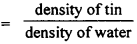= $$\frac{1.429 \times 10^3}{10^3}$$ = 1.429
Because the relative density of tin (is more than 1). Therefore, tin will sink in the water. Thus, mass of the water displaced wilt be less than that of tin.Question 11.
The volume of a piece of iron is 20cm3 and mass ¡s 156 g. What is its density?
Solution:
Mass of the iron = 156 g
Volume of the iron = 20 cm3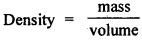= $$\frac {156}{20}$$ = 7.8g cm-3.

Do Yourself

(1) Write down the expression for the acceleration experienced by a particle on the surface of moon due to the gravitational force on moon. Find the ratio of this acceleration to that experienced by the saine particle on the surface of the earth. if the acceleration due to gravity on earth is 98 ms2 what is the acceleration of a particle on the surface of moon?
[(i) a = $$\frac{\mathrm{GM}_{\mathrm{m}}}{\mathrm{R}_{\mathrm{m}}}$$ (ii) 0.16 (iii) 1.57 ms-2]

(2) A particle is thrown up in vertical direction with a velocity of 50 ms. What will be its velocity at highest point? What is the maximum height attained by this particle? How much time will it take to reach at highest point?
[(i) v = 0 (ii) 125 m (iii) 5 s]

(3) What will be the force due to gravity on the surface of that planet whose mass and radius, both are
half than that of earth ?
[Take g = 9.8 ms2 1 [19.6 ms-2]

(4) A force of 20 N is applied on an object of weight 9.8 N. Calculate the mass of the object and the
acceleration produced.
[Take g = 9.8 ms-2] [1 kg; 20 ms-2]

(5) The mass of the earth is 6 x 1024 kg and that of the moon is 7.3 x 1o22 kg. 1f the distance between the
earth and the moon is 3.84 x 105 km, calculate the force exerted by the earth on the moon.
[19.8 x 1019 N]

(6) Calculate the force of attraction acting on person of mass 35 kg on the surface of earth. The mass of the earth is 6 x 1024 kg and radius is 6.4 x 106m.
[342 N]

(7) Find the force of attraction between two objects having mass 50 kg and 10 kg and the distance between them is 1 metre.
[3.34 x 10-8 N]

(8) The mass of earth is 6 x kg and mass of moon is 7.4 x 1022 kg. 1f the distance between the earth and the moon is 3.84 x 105 km, find the force exerted by the earth on the moon
[G = 6.7 x 10-11 Nm2 kg-2]
[22.01 x 1020N]

(9) An object is thrown up ¡n vertical direction and attains a height of 10 m. Calculate
(i) the velocity with which the object is thrown up and
(ii) time taken by the object to reach the highest point.
[u = 14 ms-1 t = 1 .43s]

(10) A boy dropped a stone from the edge of a rock of 49 m high. After is, he dropped second stone. What was the speed of second stone if both stones reached the eanh in same time ?
[ Take g = 9.8 ms-2]
[12.08 ms-1](11) By which initial velocity, the ball will be thrown up so that it can go upto a height of 40 m.
[28 ms-1] [10 kg]

(12) Find the mass of that object whose weight is 196 N.
[Take -9.8 ms-2] (10 kg]

(13) An aeroplane is flying at a height of 1000 m in horizontal direction with a velocity of 100 ms-1. A bomb is dropped from it. Calculate:
(i) the time taken by the bomb to reach earth.
(ii) the velocity before striking the ground.
(iii) the distance of target.
[(1) 14.28 s ((ii) 172.4 ms-1 ((iii) 1428.5 m]

(14) The mass of an object ¡s 10 kg. What will be its weight on the earth?
[98 N]

(15) The weight of an object is 10 N on weighing. What will be its weight on moon?
[98 N]

(16) A block of wood is kept on a table top. The mass of wooden block is 5 kg and its dimcnsions are 40 cm x 20 cm x 10cm. Find the pressure exerted by the wooden block on the table top ¡fit is made to lie on the table top with its sides of dimensions (a) 20 cm x 10 cm and (b) 40 cm x 20 cm.
[(a) 2450 Nm-2 (b) 612.5 Nm-2]

(17) The relative density of silver is 10.8 g. The density of water is 1 kgm3. What will be the density
of silver in SI unit?
[10.8 x 103 kg m3]

Essay Type Questions

Question 1.
Find the deflnition of universal gravitational constant ‘G’. Write the unit of ‘G’ In SI system.
According to Newton’s Universal Law of Gravitation,

F = $$\mathrm{G} \frac{\mathrm{M}_1 \mathrm{M}_2}{\mathrm{R}^2}$$
Or G = $$\frac{\mathrm{FR}^2}{\mathrm{M}_1 \mathrm{M}_2}$$
If M2 = M2 = 1 unit and R = 1 unit, then
G = $$\frac{F \times 1^2}{1 \times 1}=\frac{F \times 1}{1 \times 1}$$ = F
∴ Thus, universal gravitational constant is a gravitational force which is placed between the objects of unit mass which are at unit distance from each other.

SI unit of G:
G = $$\frac{\mathrm{FR}^2}{\mathrm{M}_1 \mathrm{M}_2}$$
Because G is the ratio ofF and M1 M2/R2 . Therefore, its unit depends upon the units of force, mass and distance. If mass is taken in kilogram, distance in metres and time in seconds, then unit of force is newton (N). Therefore, unit of G will be Nm-2 kg-2. The value of G found by the experiments on golden balls is:
G = 0.000,000,000,066,734 Nm2 kg2
= 6.673 x 10-11 Nm2 kg2Question 2.
State the universal law of gravitation. Derive the formula for gravitational force between two bodies. Aand B of masses M and m, placed at a distanced.$$1 \frac{1}{2}+2 \frac{1}{2}=4$$
Universal Law of Gravitation:
Every object in the universe attracts every other object with a force which is proportional to the product of their masses and inversely proportional to the square of the distance between them. The force is along the line joining the çentres of two objects.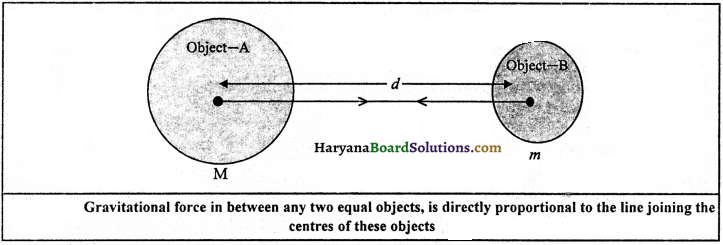Let two objects A and B of masses M and ‘m’ lies at a distance ‘d’ from each other as shown in figure. Let the force of attraction between two objects be F. According to the universal law of gravitation, the force between two objects is directly proportional to the product of their masses, that is,
F ∝ M x m …………(i)
And the force between two objects is inversely proportional to the square of the distance between them, that is,
F ∝ $$\frac{M \times m}{d^2}$$ ………..(ii)
Combining eqs. (i) and (ii), we get
F ∝ $$\frac{M \times m}{d^2}$$ or F = G $$\frac{M \times m}{d^2}$$
Where G is the constant of proportionality and is called universal gravitational constant.

Question 3.
Compare the weights of body if that are weigh on earth and moon.
The value of acceleration due to gravity of each planet (g) depends upon its mass and radius. Due to this reason, weight of an object on moon is $$\frac {1}{6}$$ th times than on the earth. Suppose the mass of object is ‘m’e. If the mass of earth is Me and its radius is Re, then weight of the object on earth (Fe).
Fe = $$\frac{\mathrm{GM}_{\mathrm{e} \mathrm{m}}}{\mathrm{R}_{\mathrm{e}}^2}$$ ………..(i)
In same way, the mass of the moon is Mm and its radius is Rm then weight of the object on moon (Fm)
Fm = $$\frac{\mathrm{GM}_{\mathrm{e}} \mathrm{m}}{\mathrm{R}_{\mathrm{e}}^2}$$ ………(ii)
Dividing eQuestion (i) by eQuestion (ii) $$\frac{\mathrm{F}_{\mathrm{e}}}{\mathrm{F}_{\mathrm{m}}}=\frac{\mathrm{GM}_{\mathrm{e}} \mathrm{m}}{\mathrm{R}_{\mathrm{e}}^2} \times \frac{\mathrm{R}_{\mathrm{m}}^2}{\mathrm{GM}_{\mathrm{m}} \times \mathrm{m}}=\frac{\mathrm{M}_{\mathrm{e}}}{\mathrm{M}_{\mathrm{m}}}\left(\frac{\mathrm{R}_{\mathrm{m}}}{\mathrm{R}_{\mathrm{e}}}\right)^2$$
∴ The mass of the earth is 100 times than that of moon, and radius is 4 times more
Me = 100 Mm and
Re = 4 Rm

By putting the value of Me and Re
$$\frac{\mathrm{F}_{\mathrm{e}}}{\mathrm{F}_{\mathrm{m}}}=\frac{\mathrm{M}_{\mathrm{e}}}{\mathrm{M}_{\mathrm{m}}}\left(\frac{\mathrm{R}_{\mathrm{m}}}{\mathrm{R}_{\mathrm{e}}}\right)^2=\frac{100 \mathrm{M}_{\mathrm{m}}}{\mathrm{M}_{\mathrm{m}}} \times\left(\frac{\mathrm{R}_{\mathrm{m}}}{4 \mathrm{R}_{\mathrm{m}}}\right)^2$$
$$\frac{\mathrm{F}_{\mathrm{e}}}{\mathrm{F}_{\mathrm{m}}}$$ = 100 x $$\frac {1}{6}$$
= 6 approx.
Fe = 6 Fm
Thus, it is clear that the mass of the object on the moon will be m, but its weight on the earth is 6 times more in compare to moon.Question 4.
Write the difference between:
(i) Gravitational force and force of gravity
(ii) Centre of mass and centre of gravity.
(i) The difference between gravitational force and force of gravity is as follows:

 Gravitational Force Force of Gravity: 1. The gravitational force is the force that arises between any two objects in the universe. 1. The force exerted between the earth and the object is called force of gravity. 2. The gravitational force exerted between two objects is proportional to the product of their masses. 2. The force of gravity is proportional to the masses of body. 3. The gravitational force exerted between the two objects is inversely proportional to the square of the distance between their centres. 3. The force of gravity is proportional to the acceleration due to gravity (g). Gravitational Force Force of Gravity:

(ii) Centre of mass and centre of gravity.
Suppose a giant body is made of many particles. Then it is possible for us to define that point where the whole of the mass of the body is concentrated. This point is called ‘centre of mass’. On the surface of earth or nearby it, where force of gravity is constant, centre of mass is only that point where the force of gravity on whole body can be experienced. In this state, the centre of mass is called centre of gravity.

Thus ‘centre of gravity’ of a body is that point, where the force of gravity is acting on the whole body as shown in fig. The centre of gravity of objects having regular size and uniform density lies on their geometrical centre. This can be balanced on a needle at the geometrical centre. For example, the centre of gravity of a spherical ball, or rectangular piece of wood or circular disc of metal is at their centre.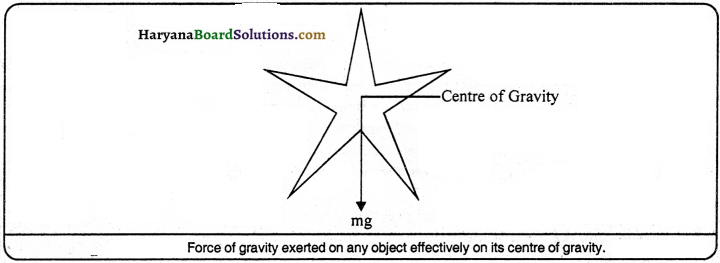Practical Work

Experiment 1.
Describe an activity to understand the motion of moon from earth. Procedure:
(i) Take a piece of thread. Tie a small stone at one end.
(it) Hold the other end of the thread and whirl it round as shown in fig.
(iii) The direction of the motion of stone will be circular motion.
(v) Again, note the direction of motion of the stone.
Before the thread is released, the stone moves in a circular path with a certain speed and changes direction at every point. The change in direction involves change in velocity or acceleration. The force that cause this acceleration and keeps the body moving along the circular path is acting towards the centre. This force is called the centripetal force.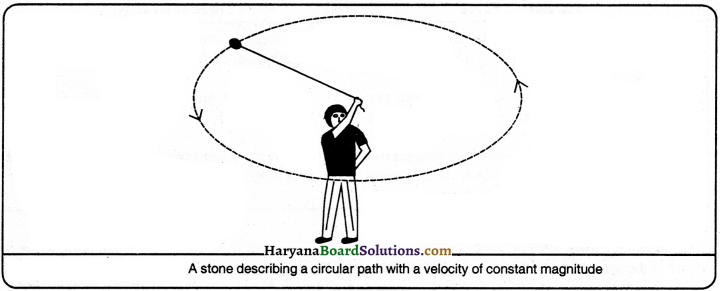Experiment 2.
Explain an activity to understand the meaning of free fall of objects.

Procedure:
(i) Take a stone.
(ii) Throw it upwards.
(iii) It reaches a certain height and then it starts falling down.
We know that the earth attracts objects towards it. This is due to force of gravity. Whenever objects fall towards the earth under this force alone, we say that the objects are in free fall.

Experiment 3.
Prove with experiment that the gravitational force between two objects of general masses is weak.

Procedure:
Take a magnet. Take it near the iron pin kept near the piece of stone. You will see that the iron pin is not attracted towards piece of stone but towards the magnet. It proves that the gravitational force between stone and pin is very weak. So the iron pin is not attracted towards stone. In fact, the gravitational force between piece of stone and iron pin is very weak as compared to magnetic force. So the iron pin attracted towards magnet but not towards piece of stone. It is concluded that the gravitational force between two objects of general masses is weak.

Experiment 4.
Explain with an experiment that why iron sinks into the water while cork floats ?

Procedure:
(i) Take a beaker filled with water.
(ii) Take an iron nail and a cork of equal mass.
(iii) Place them on the surface of water.
(iv) You will observe that the cork floats and the nail sinks.
This is due to the difference in their densities. The density of a substance is defined as the mass per unit volume. The density of cork is less than the density of water. This means that the up thrust of water on the cork is greater than the weight of the cork. So it floats.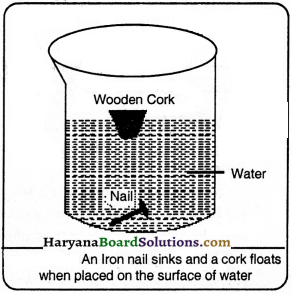Quick Review of the Chapter

1. What is the force of attraction called between the two particles of the universe ?
(A) force of gravity
(B) gravitational force
(C) centripetal force
(D) magnetic force
(B) gravitational force

2. The force exerted by the earth on an object is called :
(A) force of gravity
(B) gravitational force
(C) centripetal force
(D) magnetic force
(A) force of gravity

3. The acceleration which maintains the circular motion always directed towards the centre of circle, that is called :
(A) force of gravity
(B) gravitational force
(C) centripetal force
(D) buoyant force
(C) centripetal force4. Every object in the universe attracts every other object with a force which is proportional to the product of their masses and inversely proportional to the square of the distance between them. This law is called :
(A) universal law of gravitation
(B) law of gravity
(C) universal law of centripetal
(D) Archimedes’ principle
(A) universal law of gravitation

5. The magnitude of the gravitational force on an object on the surface of earth is :
(A) $$\frac{\mathrm{GM}_{\mathrm{e}}}{\mathrm{R}^2}$$
(B) $$\frac{\mathrm{GM}}{\mathrm{R}^2}$$
(C) $$\mathrm{GM}_{\mathrm{e}} \mathrm{m}\mathrm{R}_{\mathrm{e}^2}$$
(D) $$\frac{\mathrm{M}_{\mathrm{e} m}}{\mathrm{GR}^2}$$
(C) $$\mathrm{GM}_{\mathrm{e}} \mathrm{m}\mathrm{R}_{\mathrm{e}^2}$$

6. The value of gravitational constant is :
(A) different at each place on earth
(B) same at all planets and satellites
(C) vary from place to place
(D) all above statements are false
(B) same at all planets and satellites

7. On which part of earth the value of acceleration due to gravity (g) is maximum?
(A) on poles
(B) on equator
(C) on centre
(D) on all of them
(A) on poles

8. What is the weight of an object on moon in comparison of earth ?
(A) 6 times
(B) 3 times
(C) $$\frac {1}{6}$$ times
(D) $$\frac {1}{3}$$ times
(C) $$\frac {1}{6}$$ times9. The value of acceleration due to gravity (g) is :
(A) same at every place on earth
(B) same at all planets and satellites
(C) vary from place to place
(D) all above statements are false
(C) vary from place to place

10. If the weight of a person is 24 N on the earth, then what will be his weight on moon ?
(A) 6N
(B) 8N
(C) 4N
(D) 96 N
(C) 4 N

11. The value of acceleration due to gravity (g) is least on which part of earth ?
(A) on poles
(B) on equator
(C) on mountains
(D) on all above
(B) on equator

12. The formula of the attractive force (F) between two objects of masses ntj and m2 respectively kept at a distance of R is :
(A) $$\frac{\mathrm{Gm}_1}{\mathrm{~m}_2 \mathrm{R}^2}$$
(B) $$\frac{\mathrm{Gm}_2}{\mathrm{~m}_1 \mathrm{R}^2}$$
(C) $$\frac{\mathrm{R}^2}{\mathrm{Gm}_1 \mathrm{~m}_2}$$
(D) $$\frac{\mathrm{Gm}_1 \mathrm{~m}_2}{\mathrm{R}^2}$$
(D) $$\frac{\mathrm{Gm}_1 \mathrm{~m}_2}{\mathrm{R}^2}$$

13. The value of acceleration due to gravity (g) on the surface of earth is :
(A) 9.8 ms-2
(B) 4.9 ms-2
(C) 19.6 ms-2
(D) 29.4 ms-2
(A) 9.8 ms-2

14. The value of acceleration due to gravity (g) is zero on :
(A) poles
(B) equator
(C) centre
(D) everywhere
(C) centre15. The value of acceleration due to gravity (g) at a distance of 12800 km from the centre of earth is:
(A) 1/4 th that of earth
(B) half of the acceleration due to
(C) 4 times that of earth
(D) gravity of earth double that of earth
( A) 1/4 th that of earth

16. The distance of the moon from the earth is :
(A) 384000 m
(B) 384000 km
(C) 38400 km
(D) 38400 m
(B) 384000 km

17. The quantity of matter present in the body is called :
(A) weight
(B) mass
(C) pressure
(D) thrust
(B) mass

18. The weight of an object is zero at:
(A) poles
(B) equator
(C) mountains
(D) centre of earth
(D) centre of earth

19. What is the relation among u, y, g and t?
(A) v = u + gt
(B) v + u = gt
(C) v + gt = u
(D) $$\frac {v}{g}$$ = u + t
(A) v = u + gt20. The distance of sun from earth is approximately:
(A) 1.5 x 1011 m
(B) 1.5 x 1011 km
(C) 1.5 x 1011 cm
(D) 1.5 x 1011 mm
(A) 1.5 x 1011 m

21. By which symbol pascal is denoted?
(A) Pa
(B) Ps
(C) Pc
(D) P
(A) Pa

22. On what angle a player throws ball with horizontal line so the ball falls at maximum distance?
(A) 900
(B) 0°
(C) 45°
(D) 600
(C) 45°

23. The free fall means:
(A) the objects fàlling towards earth
(B) the objects going upward from the earth
(C) the objects moving parallel to earth
(D) none of these
(A) the objects falling towards earth

24. SI unit of pressure is:
(A) Newton
(B) kilogram
(C) metre
(D) Pascal
(D) Pascal

25. What will be the produced pressure 11300 n force is applied on the area of 20m2?
(A) 6000 Pa
(B) 3OPa
(C) 15 Pa
(D) 7.5 Pa
(C) 15 Pa26. It is difficult to hold a school bag having a strap made of a thin and strong string, because thrust affects:
(A) large area
(B) more area
(C) small area
(D) longer area